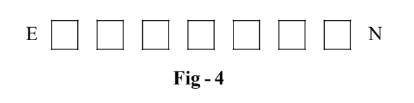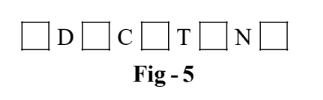# Introduction To Permutations

Go back to  'P & C, Binomial Theorem'

We now formally study the concept of permutations, by generalising the last example in the preceding section.

The fundamental issue in Permutations is the arrangement of things. In the last example, we had 6 letters and 5 places where we could arrange (5 of) those 6 letters, We calculated that there are 720 ways of arranging those 6 letters, taken 5 at a time. In mathematical terminology, we calculated as 720 the number of permutations (arrangements) of 6 letters taken 5 at a time.

Let us generalise this: Suppose we have n people. It we had n seats available to seat these people, the total number of ways to do so would be (by the logic discussed in the preceding section) $$n \times (n - 1) \times (n - 2) \times ... \times 1$$ . This quantity is denoted by  $$n!$$.

$n! = n \times (n - 1) \times (n - 2) \times ... \times 1$

Suppose now that we have only r seats, where r < n. The total number of ways now would be $$n \times (n - 1) \times (n - 2) \times ... \times (n - r + 1)$$ . This is the number of ways of permuting n things, taken r at a time, and the notation used for this number is $${}^n{P_r}$$ . Thus :

\begin{align}&{}^n{P_r} = n \times (n - 1) \times (n - 2) \times ... \times (n - r + 1)\\\\&\quad\;=\frac{{n \times (n - 1) \times (n - 2) \times ... \times (n - r + 1) \times (n - r) \times ...1}}{{(n - r) \times ... \times 1}}\\&\quad\;=\frac{{n!}}{{(n - r)!}}\end{align}

Thus, using the fundamental principle of counting, you see that we’ve been able to calculate the value of   $${}^n{P_r}$$ .

Finally, note that $${}^n{P_n} = n!$$

Lets apply this discussion to an example:

Example – 1

Find the number of permutations of the word EDUCATION which

 (a)  consist of all the letters of this word (b)  start with E and end with N (c)  contain the string “CAT” (d)  have the letters C, A and T occurring together (e)  start and end with vowels (f)   have no two vowels occurring together.

Solution:   (a) We have 9 letters and we want to permute all of them. The required number of arrangements would be $${}^9{P_9} = 9!$$

(b) Fix E at the start and N at the end of the wordWe now have 7 places which we need to fill using the remaining 7 letters. The number of such permutations will  be $${}^7{P_7} = 7!$$  .

(c)  We want all those permutations in which the string “CAT” occurs. Let us treat “CAT” as a single letter/object since this is what we want - we want “CAT” to appear as a single entity. We now have the following objects (letters) which we need to permute:

$\text{E D U “CAT ” I O N}$

These are in total 7 objects. Thus, they can be permuted in   $${}^7{P_7} = 7!$$  ways. This is the number of  permutations that contain the string “CAT”.

(d) We now need the permutations which contain the letters C, A and T occurring together. This means that they can occur together in any order. There are 3! = 6 ways in which C, A and T can be arranged among themselves, namely CAT, CTA, ACT, ATC, TAC, and TCA.

Now consider from the previous part all those permutations in which the string “CAT” occurs. These are 7! in number. Corresponding to each such permutation, we can have 6 permutations in which the constraint is that C, A and T occur together. This is because the string “CAT” can itself be permuted in 6 ways as described above. For example, consider the permutation “EDUCATION”; to this permutation will correspond 6 permutations in which C, A and T occur together:

$EDU\fbox{CAT}ION\rightarrow{{\,\,\,\,\,\,\,\,\,\,\,\,\,\,\,\,\,\,\,}}\left\{ \begin{gathered} EDU\fbox{CAT}ION \hfill \\ EDU\fbox{CTA}ION \hfill \\EDU\fbox{ACT}ION \hfill \\EDU\fbox{ATC}ION \hfill \\EDU\fbox{TAC}ION \hfill \\EDU\fbox{TCA}ION \hfill \\\end{gathered} \right\}$

The required number of permutations is therefore $${\text{7!} \times \text{3!}}$$ .

(e)  In the word EDUCATION, there are a total of 5 vowels. We want to have the starting and ending letters of our permutations as vowels. Let us first arrange the starting and ending letters and then fill in the rest of the 7 places. Out of the 5 vowels available to us, the first and the last place can be filled in $${}^5{P_2}$$  places. Once we’ve fixed the first and the last letters, the remaining 7 places can be filled in $${}^7{P_7} = 7!$$   ways. Thus, the total number of required permutations is $${}^5{P_2} \times 7!$$ .

(f)  There are 5 vowels and 4 consonants in the word EDUCATION. We require permutations in which no two vowels occur together. We can ensure such permutations by first fixing the 4 consonants and then arranging the 5 vowels in the 5 possible places that arise as depicted in the following figure.Convince yourself that any arrangement of the 5 vowels in the 5 blank spaces above will correspond to a permutation with no two vowels together.

The number of ways of arranging the 4 consonants is $${}^{\rm{4}}{P_4} = 4!$$ . After the consonants have been arranged, the number of ways of arranging the 5 vowels in the 5 blank spaces as depicted in Fig.5 is 5!. Thus, the required number of permutations is 4! × 5!

It would be a good exercise for you to construct more of such examples on your own and solve them. You can take an arbitrary word and find the number of permutations of that word, that satisfy any particular condition that you can think of.

Learn from the best math teachers and top your exams

• Live one on one classroom and doubt clearing
• Practice worksheets in and after class for conceptual clarity
• Personalized curriculum to keep up with school